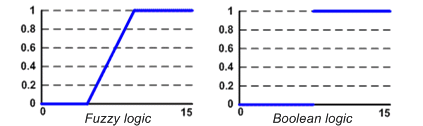# Fuzzy logic

Fuzzy logic was developed by Lotfi A. Zadeh in the 1960s in order to provide mathematical rules and functions which permitted natural language queries. Fuzzy logic provides a means of calculating intermediate values between absolute true and absolute false with resulting values ranging between 0.0 and 1.0.  Fuzzy logic calculates the shades of gray between black/white and true/false.

Fuzzy logic is a super set of conventional (or Boolean) logic and contains similarities and differences with Boolean logic. Fuzzy logic is similar to Boolean logic, in that Boolean logic results are returned by fuzzy logic operations when all fuzzy memberships are restricted to 0 and 1. Fuzzy logic differs from Boolean logic in that it is permissive of natural language queries and is more like human thinking; it is based on degrees of truth.A fuzzy set is a set whose elements have degrees of membership. That is, a member of a set can be full member (100% membership status) or a partial member (eg. less than 100% membership and greater than 0% membership). To fully understand fuzzy sets, one must first understand traditional sets.

Fuzzy Logic deals with those imprecise conditions about which a true/false value cannot be determined. Much of this has to do with the vagueness and ambiguity that can be found in everyday life. For example, the question:Is it HOT outside?probably would lead to a variety of responses from those asked. These are often labeled as subjective responses, where no one answer is exact. Subjective responses are relative to an individual's experience and knowledge. Human beings are able to exert this higher level of abstraction during the thought process. For this reason, Fuzzy Logic has been compared to the human decision making process. Conventional Logic (and computing systems for that matter) are by nature related to the Boolean Conditions (true/false). What Fuzzy Logic attempts to encompass is that area where a partial truth can be established, that is a gradient within the true/false realm.